# A short proof for Nesterov’s momentum

Yesterday I posted the following picture on Twitter and it quickly became my most visible tweet ever (by far):I thought this would be a good opportunity to revisit the proof of Nesterov’s momentum, especially since as it turns out I really don’t like the way I described it back in 2013 (and to this day the latter post also remains my most visible post ever…). So here we go, for what is hopefully a short and intuitive proof of theconvergence rate for Nesterov’s momentum (disclaimer: this proof is merely a rearranging of well-known calculations, nothing new is going on here).

We assume thatis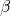-smooth convex function, and we takein the gradient step. The momentum term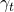will be set to a very particular value, which comes out naturally in the proof.

The two basic inequalities

Let us denoteand(note that). Now let us write our favorite inequalities (using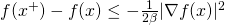and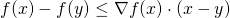):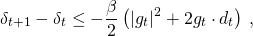and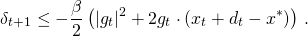On the way to a telescopic sum

Recall now that, so it would be nice to somehow combine the two above inequalities to obtain a telescopic sum thanks to this simple formula. Let us try to take a convex combination of the two inequalities. In fact it will be slightly more elegant if we use the coefficienton the second inequality, so let us do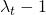times the first inequality plustimes the second inequality. We obtain an inequality whose right hand side is given bytimesRecall that our objective is to obtain a telescopic sum, and at this point we still have flexibility both to chooseand. What we would like to have is:Observe that (since) the right hand side can be written as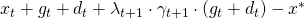, and thus we see that we simply need to have: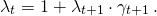Setting the parameters and concluding the proof

Writing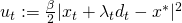we now obtain as a result of the combination of our two starting inequalities:It only remains to selectsuch that(i.e., roughlyis of order) so that by summing the previous inequality one obtainswhich is exactly therate we were looking for.

This entry was posted in Optimization. Bookmark the permalink.

## 11 Responses to "A short proof for Nesterov’s momentum"

•••••••••••